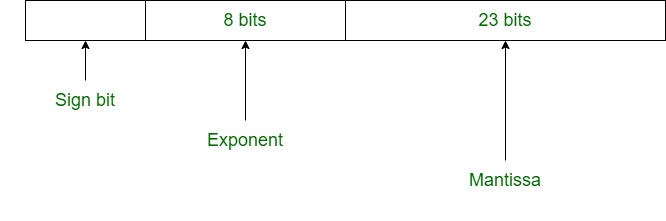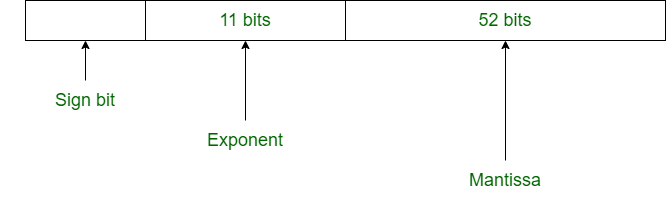# Difference between Single Precision and Double Precision

• Difficulty Level : Easy
• Last Updated : 09 May, 2020

According to IEEE standard, floating-point number is represented in two ways:

 Precision Base Sign Exponent Significand Single precision 2 1 8 23+1 Double precision 2 1 11 52+1

1. Single Precision:
Single Precision is a format proposed by IEEE for representation of floating-point number. It occupies 32 bits in computer memory.2. Double Precision:
Double Precision is also a format given by IEEE for representation of floating-point number. It occupies 64 bits in computer memory.Difference between Single and Double Precision:

SINGLE PRECISIONDOUBLE PRECISION
In single precision, 32 bits are used to represent floating-point number.In double precision, 64 bits are used to represent floating-point number.
It uses 8 bits for exponent.It uses 11 bits for exponent.
In single precision, 23 bits are used for mantissa.In double precision, 52 bits are used for mantissa.
Bias number is 127.Bias number is 1023.
Range of numbers in single precision : 2^(-126) to 2^(+127)Range of numbers in double precision : 2^(-1022) to 2^(+1023)
This is used where precision matters less.This is used where precision matters more.
It is used for wide representation.It is used for minimization of approximation.
It is used in simple programs like games.It is used in complex programs like scientific calculator.
This is called binary32.This is called binary64.

Please refer Floating Point Representation for details.

My Personal Notes arrow_drop_up